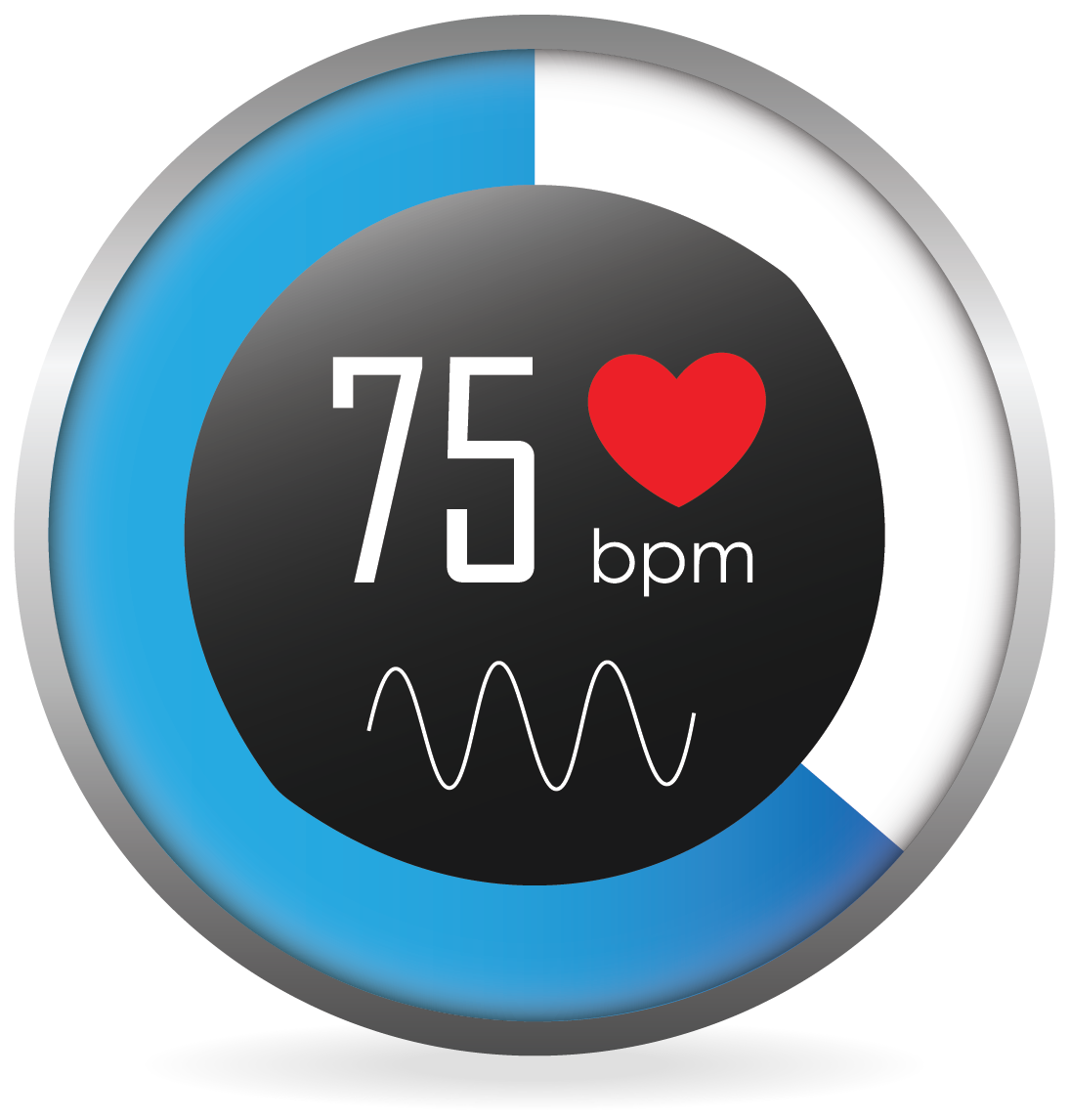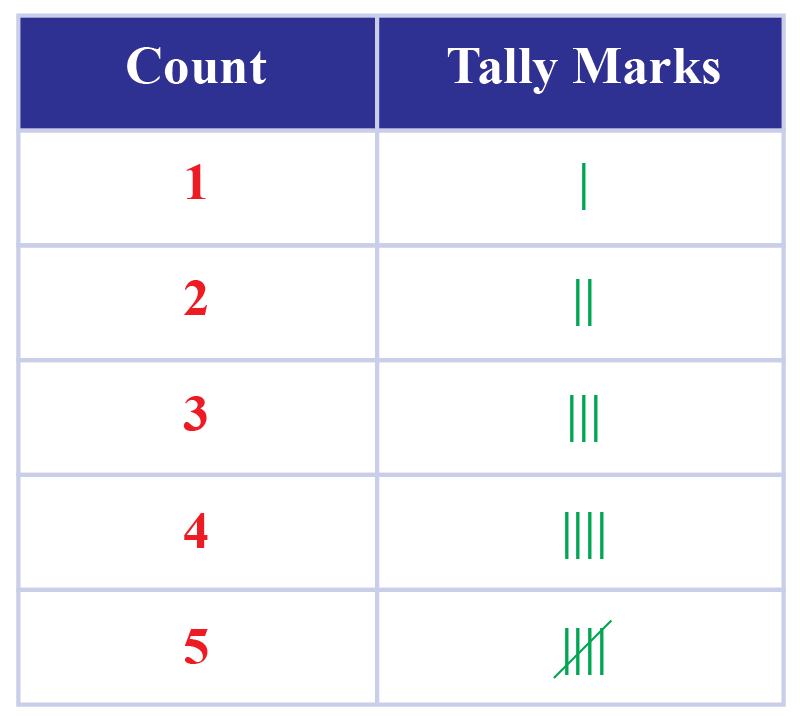# Frequency Distribution Table

Frequency Distribution Table

In this lesson, you will be introduced to the concept of frequency distribution. Right from representing class performances in schools to representing statistical data in other fields, there are several applications of frequency distribution tables and graphs. When it comes to finance, frequency distributions are used by traders to make note of the prices and other details and also identify trends. Therefore, it is an important concept in Math.

You can get a brief idea about frequency distribution by exploring the simulation shown above. As you go through this lesson, you will learn how to create a frequency distribution table and the formulas and graphs related to frequency distributions.

Let's begin!

## Lesson Plan

 1 What Is Frequency Distribution? 2 Thinking Out of the Box! 3 Tips and Tricks 4 Important Notes on Frequency Distribution Table 5 Solved Examples on Frequency Distribution Table 6 Interactive Questions on Frequency Distribution Table

## Frequency

Do you know the meaning of "frequency?"

Frequency indicates how often something occurs.

For example, your heartbeat is 72 heartbeats/min under normal conditions.Frequency corresponds to the number of times a value occurs.

Here are a few examples.

Annie takes music class once every week.

Thus, frequency = 1

Annie takes dance classes as per the following schedule: 1 lesson each on Mondays, Wednesdays, and Fridays.

Thus, frequency per week = 3

## What Is Frequency Distribution?

In our day-to-day lives, we come across a lot of information in the form of numerical figures, tables, graphs, etc.

This information could be marks scored by students, temperatures of different cities, points scored in matches, etc.

The information that is collected is called data.

Once the data is collected, we have to represent it in a meaningful manner so that it can be easily understood.

The data should be organized in such a way that all its features are summarized in a table and a glance of it should be enough to understand the data.

How do we go about doing this?

The frequency distribution is one of the ways to organize data.

We will learn all about it here.

## Frequency Distribution Calculator

Let's first explore the frequency distribution calculator below.

Enter the data and observe how the frequency distribution table is created.

Frequency distribution table calculators such as the one above save a lot of time when tabulating the data.

It makes calculations easy and leads to the organization of data in seconds.

## Frequency Distribution Table

A frequency distribution table is a way to organize data so that it makes the data more meaningful.

Here's a frequency distribution table example for you to understand this concept better.

Jane is fond of playing games with dice.She throws the dice and notes the observations each time.

These are her observations:

4, 6, 1, 2, 2, 5, 6, 6, 5, 4, 2, 3

To know the exact number of times she got each digit (1, 2, 3, 4, 5, 6) as the outcome, she classifies them into categories.

An easy way is to use tally marks.

Note that a diagonal line across 4 vertical lines counts as 5Outcomes Tally Marks Frequency
1 1
2 I I I 3
3 I 1
4 I I 2
5 I I 2
6 I I I 3

The table above is known as a frequency distribution table.

You can observe that all the data that was gathered has been organized under two columns.

Thus, a frequency distribution table is a chart summarizing the values and their frequencies.

In other words, it is a tool to organize data.

This makes it easy for us to understand the given set of information.

Thus, frequency distribution in statistics helps us condense data in a simpler form so that it is easy for us to observe its features at a glance.Think Tank
• When dealing with quantitative data, we divide them into categories or class intervals. Does the same follow for qualitative data as well?

## How Do You Construct a Frequency Distribution Table?

Let's look at an example.

Meet Ms. Jennifer and her assistant, Mr. Thomas.

Ms. Jennifer is a teacher.

She wants to look at the marks obtained by the students of her class in the last exam.

She does not have the time to go through each test paper individually to see the marks.

Thus, she asks Mr. Thomas to organize the data in a table so that it is easier for her to look at everyone's marks together.Ms. Jennifer suggests using a frequency distribution table to organize the data, so as to get a better picture of the data rather than using a simple list.

Using a frequency distribution table here is a good way to present the data as it will show Ms. Jennifer all the students' marks in one table.

But how can a frequency distribution table be created?

Mr. Thomas works hard to put together all the data.

The following table shows the test scores of 20 students, i.e., for one class.

Marks obtained in the test Number of students (Frequency)
9 1
11 4
13 1
18 1
20 1
21 2
22 1
23 3
25 1
26 3
29 1
30 1
Total 20

A frequency distribution table such as the one above is called an ungrouped frequency distribution table.

It is the representation of ungrouped data and is typically used when you have a smaller data set.

Imagine how difficult it would be to create a similar table if you have a large number of observations, for example, the marks of students of three classes.

The table we will obtain will be quite lengthy and the data will be confusing.

Hence, in such cases, we form class intervals to tally the frequency for the data that belongs to that specific class interval.

To make a frequency distribution table, first write the categories or class intervals in one column.

Next, tally the numbers in each category based on the number of times it appears.

Finally, write the frequency in the final column.

The frequency is the total.

Marks obtained in the test Number of students (Frequency)
0 - 5 3
5 - 10 11
10 - 15 12
15 - 20 19
20 - 25 7
25 - 30 8
Total 60

A frequency distribution table such as the one above is called a grouped frequency distribution table.Tips and Tricks
1. The frequency distribution formula is as follows: Divide the number of categories/values into intervals, then count the number of results in each interval. These number of times or occurrences represent frequency.

2. There are two easy ways to create a frequency distribution table in excel: COUNTIFS Function and FREQUENCY Function.

3. R is a widely-used statistical programming language. It is a tool for data processing and analysis. When you have a limited number of values (different values) in R, a quick summary of the data can be found by calculating a frequency table.

## How Do You Describe a Frequency Distribution Table?

A frequency distribution table is a chart that summarizes all the data under two columns, values, and their frequency.

We have already learned the construction of the frequency distribution table in the section above.

It has two or three columns.

The first column lists all the various outcomes as individual values or in the form of class intervals, depending upon the size of the data set.

The second column includes the tally marks of each outcome.

The third column lists the frequency of each outcome.

Also, the second column can be optional.

More Important Topics
Numbers
Algebra
Geometry
Measurement
Money
Data
Trigonometry
Calculus